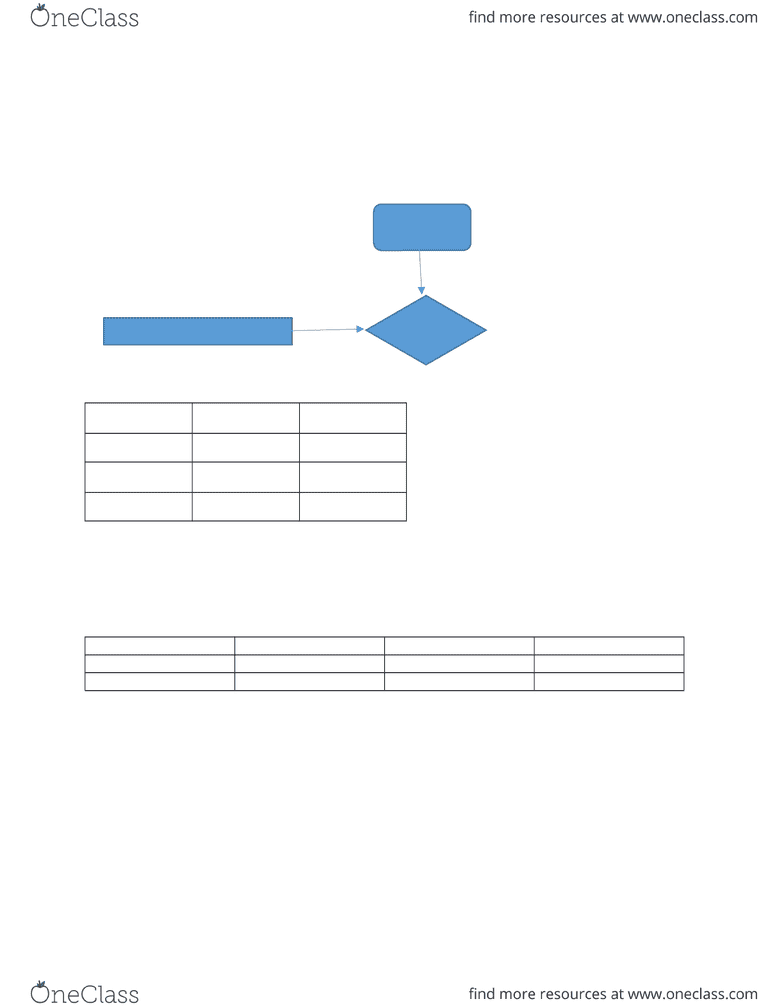Class Notes (1,100,000)
CA (620,000)
UTSC (30,000)
MGO (20)
Lecture 1

# MGOC 10 Lecture 1: Week 10

Department
Management (MGO)
Course Code
MGOC10H3
Professor
Igor Averbakh
Lecture
1

This preview shows page 1. to view the full 4 pages of the document.Week 10
Queson 13-1
Decion: type of service chance event:
Demand consequence prot
Max Prot Min Prot
Full 960 -490
Discount 670 320
Probability 0.7 0.3
Opmisc Approach: Full Price
Conservave Approach: Discount
Opportunity Loss ( Regret Table)
High Demand Low Demand Max Regret
Full Price 0 810 810
Discount 290 0 290
Min Max Regret Approach:
a) Expected Value ( full) =0.7*960+[0.3*(-490)]=525
Expected Value(Discount)=0.7*670+(0.3*3200=565
b) If Probability of strong demand =0.8,
Expected value of full=0.8*960+0.2*(-490)=670
Expected value of discount=0.8*670+(0.2*320)=600
c) Let P=probability of strong demand
Expected value of full=960p+(-490)(1-p)=1450p-490
Expected value of discount=670p+(1-p)*320=350p+320
1450P-490=350p+320
P=0.7564
If p>0.7564,discount price is beBer
Demand
Prot
Type of Service
###### You're Reading a Preview

Unlock to view full version